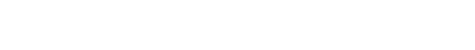# Find the Mean of the Probability Distribution / Binomial

Contents:

## How to find the mean of a probability distribution

Watch the video for an example:

Watch this video on YouTube.

## How to find the mean of the probability distribution : OverviewA normal distribution curve is one kind of probability distribution.
Finding the mean of a probability distribution is easy in probability and statistics—if you know how. This how to will guide you through a few simple steps necessary to find the mean of the probability distribution or binomial distribution. You’ll often find these types of questions in textbook chapters on binomial probability distributions. The binomial distribution is just a simple trial where there are two outcomes: success or failure. For example, if you are counting how many times you draw an Ace from a deck of cards, you could assign “Success” to “Drawing an Ace” and “Failure” to drawing any other card. You can find the mean of the probability distribution by creating a probability table.

## How to find the mean of the probability distribution: Steps

Example question: “A grocery store has determined that in crates of tomatoes,  95% carry no rotten tomatoes, 2% carry one rotten tomato, 2% carry two rotten tomatoes, and 1% carry three rotten tomatoes.  Find the mean number of rotten tomatoes in the crates.”

• Step 1: Convert all the percentages to decimal probabilities. For example:
95% = .95
2% = .02
2% = .02
1% = .01
• Step 2: Construct a probability distribution table. (If you don’t know how to do this, see how to construct a probability distribution).)• Step 3:  Multiply the values in each column. (In other words, multiply each value of X by each probability P(X).)
Referring to our probability distribution table:
0 × .95 = 0
1 × .02  = .02
2 × .02  = .04
3 × .01 = .03
• Step 4: Add the results from step 3 together.
0 + .02 + .04 + .03 = .09 is the mean.

You’re done finding the mean for a probability distribution!

Need help with a homework question? Check out our tutoring page!

## Mean of Binomial DistributionA coin toss is a simple binomial experiment.
A binomial distribution represents the results from a simple experiment where there is “success” or “failure.” For example, if you are polling voters to see who is voting Democrat, the voters that say they will vote Democrat is a “success” and anything else is a failure. One of the simplest binomial experiments you can perform is a coin toss, where “heads” could equal “success” and “tails” could equal “failure.”

The mean of binomial distribution is much like the mean of anything else. It answers the question “If you perform this experiment many times, what’s the likely (the average) result?.

## Formula for Mean of Binomial Distribution

The formula for the mean of binomial distribution is:
μ = n *p

Where “n” is the number of trials and “p” is the probability of success.

For example: if you tossed a coin 10 times to see how many heads come up, your probability is .5 (i.e. you have a 50 percent chance of getting a heads and 50 percent chance of a tails) and “n” is how many trials — 10. Therefore, the mean of this particular binomial distribution is:
10 * .5 = 5.

This makes sense: if you toss a coin ten times you would expect heads to show up on average, 5 times.

### Mean for a Binomial Distribution on the TI-83

Sample problem: Find the mean for a binomial distribution with n = 5 and p = 0.12.

Again, the TI 83 doesn’t have a function for this. But if you know the formula (n*p), it’s pretty easy to enter it on the home screen.
Step 1: Multiply n by p.
5 * .12 ENTER
=.6

Hey, that was easy!

### Something to think about:

You may be wondering why it was so easy to calculate the mean. After all being asked to “calculate the mean for a binomial distribution” sounds scary. If you think about what a mean (or average) is, then you’ll see why it was so easy. In the sample question, n = 5 and p = 0.12. What is “n”? That’s the number of items. So imagine a list of 5 items with a certain score:
1 = 0.12
2 = 0.12
3 = 0.12
4 = 0.12
5 = 0.12
If you were asked to find the average score for those five items, you wouldn’t even have to do the math: it’s just 0.12, right? Finding the mean for a binomial distribution is just a little different: you add up all of the probabilities (0.12 + 0.12 + 0.12 + 0.12 + 0.12). Or a faster way, just multiply n by p.

Check out our Youtube channel for more help and stats tips!

## References

Agresti A. (1990) Categorical Data Analysis. John Wiley and Sons, New York.
Vogt, W.P. (2005). Dictionary of Statistics & Methodology: A Nontechnical Guide for the Social Sciences. SAGE.
Lindstrom, D. (2010). Schaum’s Easy Outline of Statistics, Second Edition (Schaum’s Easy Outlines) 2nd Edition. McGraw-Hill Education

CITE THIS AS:
Stephanie Glen. "Find the Mean of the Probability Distribution / Binomial" From StatisticsHowTo.com: Elementary Statistics for the rest of us! https://www.statisticshowto.com/probability-and-statistics/binomial-theorem/find-the-mean-of-the-probability-distribution-binomial/
---------------------------------------------------------------------------Need help with a homework or test question? With Chegg Study, you can get step-by-step solutions to your questions from an expert in the field. Your first 30 minutes with a Chegg tutor is free!